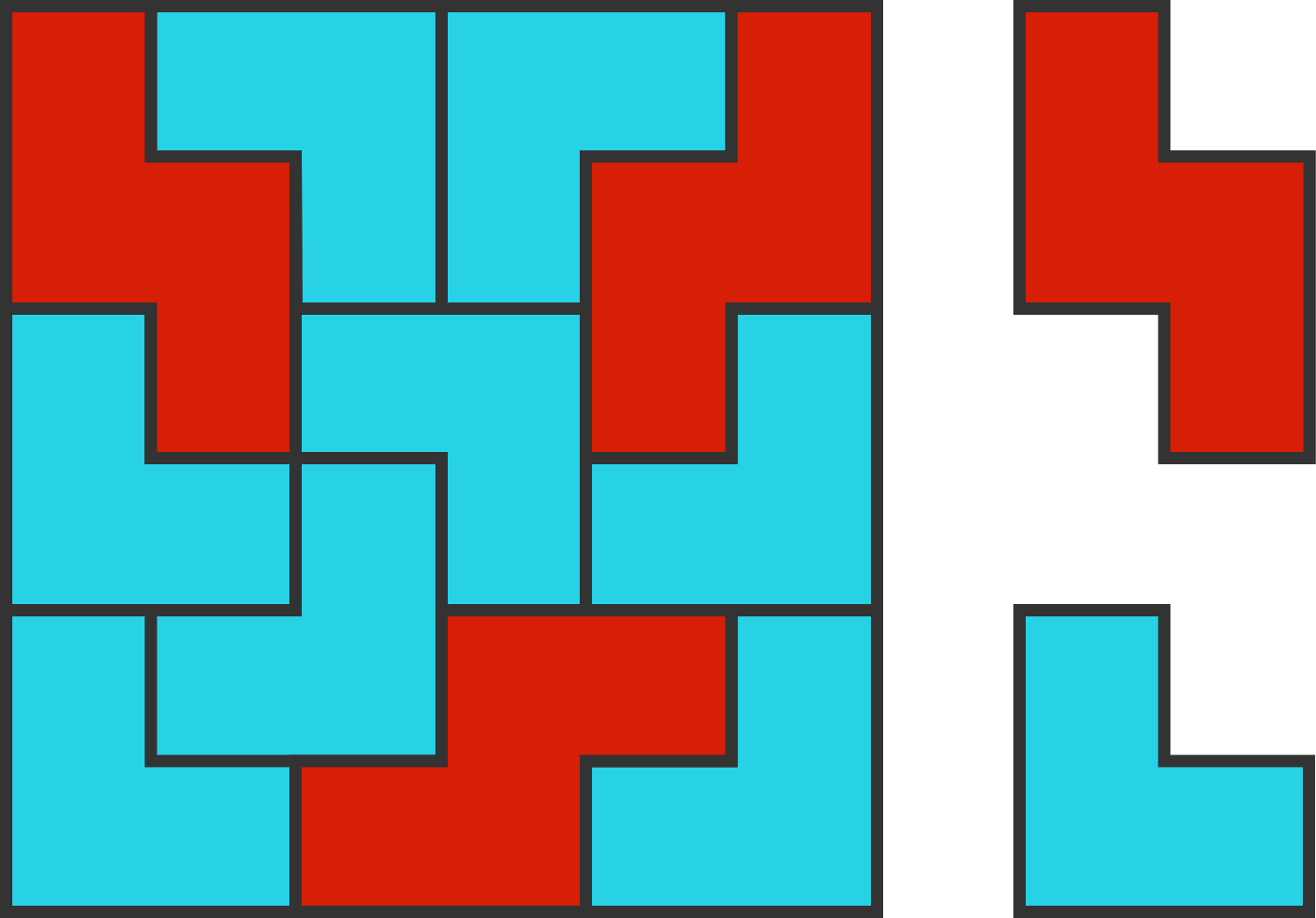# True Color

Geometry Level 2This $6 \times 6$ square has three Z-tiles (red) and eight V-tiles (blue).

You want to build a $9 \times 9$ square using these two types of tiles, both of which can be rotated and reflected.

What is the maximum number of Z-tiles you can use?

×